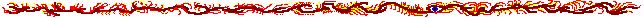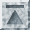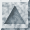# heat.cc [plain] [printer]

```#include <stdio.h>
#include <magic/applic.h>
#include <magic/Math.h>

#include <mpi++.h>
#include <mpe++.h>

#include "fdgrid.h"

class HeatGridSegment : public FDGridSegment {
public:
HeatGridSegment (int N, MPIInstance& mpi, double omega, int updatefreq)
: FDGridSegment (N, mpi), mMPE (mpi.world(), 0, 0, N, N, NULL) {
MPE_Color tmpColor;
mMPE.makeColorArray (tmpColor, 64);
// Reverse the color order (we want blue to be cold and red to be hot).
for (int i=0; i<64; i++)
mColors[i] = tmpColor[63-i];

mOmega = omega;
mUpdateFreq = updatefreq;
}

virtual double  initPoint       (int row, int col) const {
// Left, upper, and right edges have temperature 100 degrees
if (row<0 || col<0 || col>=N())
return 100.0;
else // Everywhere else the temperature is 0.0 degrees
return 0.0;
}

virtual double  compute         (int r, int c) const {
double result = mOmega/4*(value(r-1,c)+value(r+1,c)+value(r,c-1)+value(r,c+1))
+ (1-mOmega)*value(r,c);

// Plot the point to the picture every 10th cycle
if (!(cycle()%mUpdateFreq))
mMPE.drawPoint (c, r, mColors[int(63.0*fabs(result)/100.0)%64]);
return result;
}

virtual void    endOfCycle      () {
// Draw the picture every 10th cycle
if (!(cycle()%mUpdateFreq)) {
mMPE.update (); // Finalize drawing

// Check if the mouse has been pressed every 10th
// cycle. We do this only with process 0, because the MPE
// doesn't seem to like the others to call the mouse
// routines.
if (mpi().world().getRank()==0) {
int button;
int x, y;

// Check for left mouse button
if (mMPE.getMousePressed (MPE_BUTTON1, &x, &y))
try { printf ("Temperature at point (%d,%d) is %g degrees.\n",
x, y, value (y,x)); } catch (...) {}

// Check for middle mouse button. This doesn't work
// for some reason. The MPE seems to be able to take
// all mouse presses as BUTTON1.
if (mMPE.getMousePressed (MPE_BUTTON2))
printf ("Iteration %d.\n", cycle());

// Thus we print the cycle here.
printf ("Iteration %d.\n", cycle());
fflush (stdout);
}
}
}

private:
mutable MPEWindow   mMPE;
MPE_Color           mColors;
double              mOmega;
int                 mUpdateFreq;
};

Main () {
MPIInstance mpi (mArgc, mArgv);

HeatGridSegment segment (150, mpi, 1.2, 10);
segment.execute (100000, 0.001);
}
```Generated with (Python version) of tab4.cgi by Marko Grönroos (magi@iki.fi), 1999